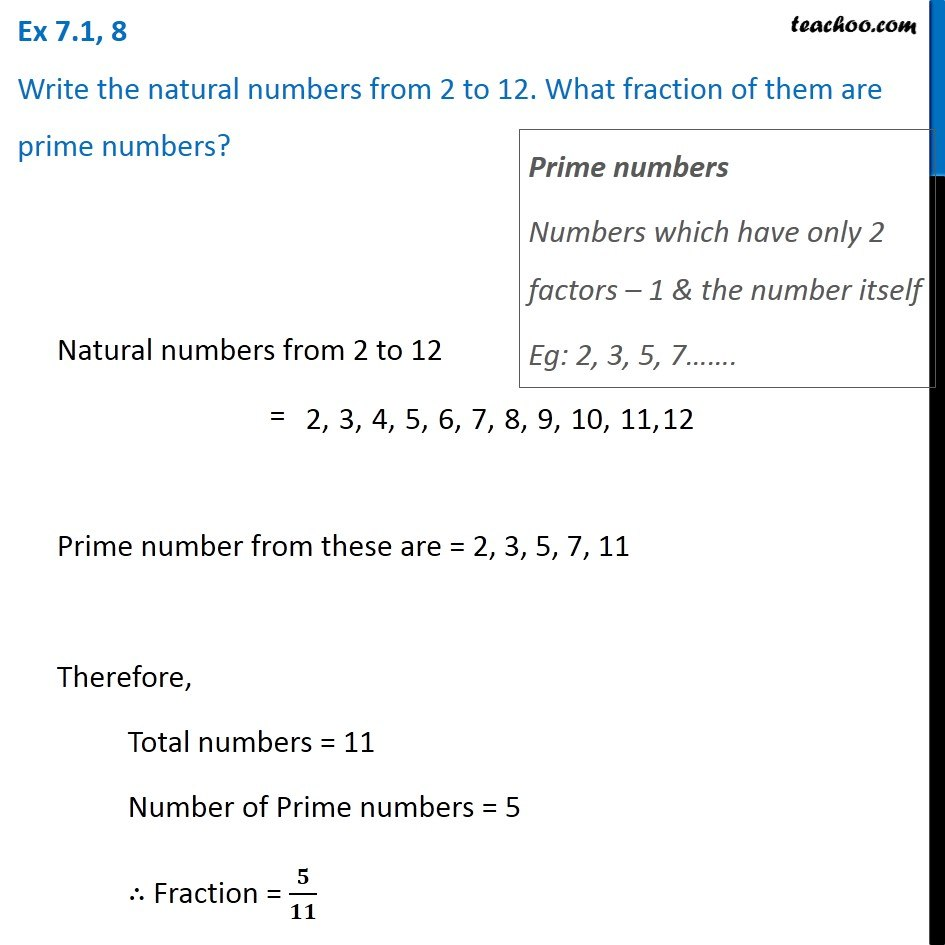Ex 7.1

Chapter 7 Class 6 Fractions
Serial order wiseGet live Maths 1-on-1 Classs - Class 6 to 12

### Transcript

Ex 7.1, 8 Write the natural numbers from 2 to 12. What fraction of them are prime numbers? Natural numbers from 2 to 12 = Prime number from these are = 2, 3, 5, 7, 11 Therefore, Total numbers = 11 Number of Prime numbers = 5 ∴ Fraction = 𝟓/𝟏𝟏 Prime numbers Numbers which have only 2 factors – 1 & the number itself Eg: 2, 3, 5, 7…….